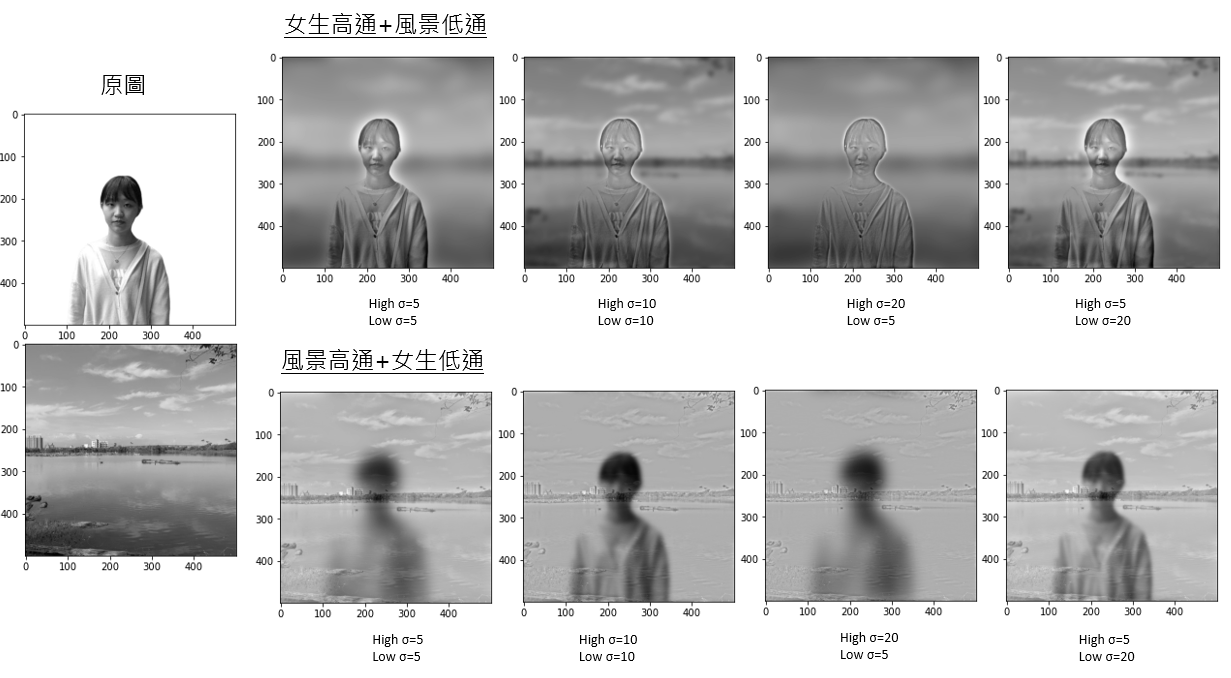## ¶ 1. 簡介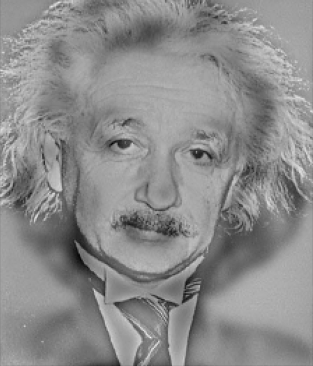## ¶ 2. 原理

### ¶ 2.1 概念(source from Wikipedia)

### ¶ 2.2 數學

\begin{align} R_{ij} &= \sum_{u,v} H_{i-u, j-v} F_{u,v} \\ \mathbf R &= \mathbf H ** \mathbf F \end{align}

$$g(i,j) = EXP({ -{ {(x-i)^2+(y-j)^2} \over{ 2 \sigma ^2}} })$$

### ¶ 2.3 濾鏡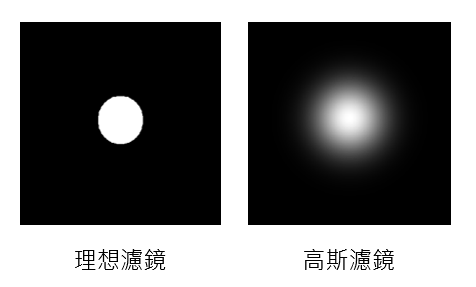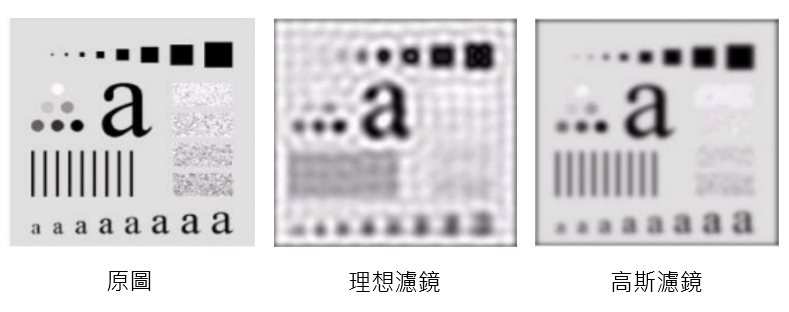## ¶ 3. 實現

### ¶ 3.1 細部介紹

def makeGaussianFilter(n_row, n_col, sigma, highPass=True):

center_x = int(n_row/2) + 1 if n_row % 2 == 1 else int(n_row/2)
center_y = int(n_col/2) + 1 if n_col % 2 == 1 else int(n_col/2)

def gaussian(i, j):
coefficient = math.exp(-1.0 * ((i - center_x) **
2 + (j - center_y)**2) / (2 * sigma**2))
return 1 - coefficient if highPass else coefficient

return numpy.array(
[[gaussian(i, j) for j in range(n_col)] for i in range(n_row)])


def filter(img, sigma, highPass):
# 計算圖片的離散傅立葉
shiftedDFT = fftshift(fft2(img))
# 將 F 乘上濾鏡 H(u, v)
filteredDFT = shiftedDFT * \
makeGaussianFilter(
image.shape, image.shape, sigma, highPass=isHigh)
# 反傅立葉轉換
res = ifft2(ifftshift(filteredDFT))

return numpy.real(res)


shiftedDFT 就是將圖片先做離算傅立葉轉換 (fft)，然後將做平移將頻率 0 置中 (fftshift)。

def hybrid_img(high_img, low_img, sigma_h, sigma_l):
res = filter(high_img, sigma_h, isHigh=True) + \
filter(low_img, sigma_l, isHigh=False)
return res


### ¶ 3.2 完整程式碼

import numpy
from numpy.fft import fft2, ifft2, fftshift, ifftshift
import math
import imageio
import matplotlib.pyplot as plt
from skimage.transform import resize

def makeGaussianFilter(n_row, n_col, sigma, highPass=True):

center_x = int(n_row/2) + 1 if n_row % 2 == 1 else int(n_row/2)
center_y = int(n_col/2) + 1 if n_col % 2 == 1 else int(n_col/2)

def gaussian(i, j):
coefficient = math.exp(-1.0 * ((i - center_x) **
2 + (j - center_y)**2) / (2 * sigma**2))
return 1 - coefficient if highPass else coefficient

return numpy.array([[gaussian(i, j) for j in range(n_col)] for i in range(n_row)])

def filter(image, sigma, isHigh):
shiftedDFT = fftshift(fft2(image))
filteredDFT = shiftedDFT * \
makeGaussianFilter(
image.shape, image.shape, sigma, highPass=isHigh)
res = ifft2(ifftshift(filteredDFT))

return numpy.real(res)

def hybrid_img(high_img, low_img, sigma_h, sigma_l):
res = filter(high_img, sigma_h, isHigh=True) + \
filter(low_img, sigma_l, isHigh=False)
return res

resize(img2, (img1.shape, img1.shape)) # 兩張圖要一樣大

plt.show(plt.imshow(hybrid_img(img1, img2, 10, 10), cmap='gray'))


## ¶ 4. 結果呈現

### ¶ 4.1 高斯過濾的低通和高通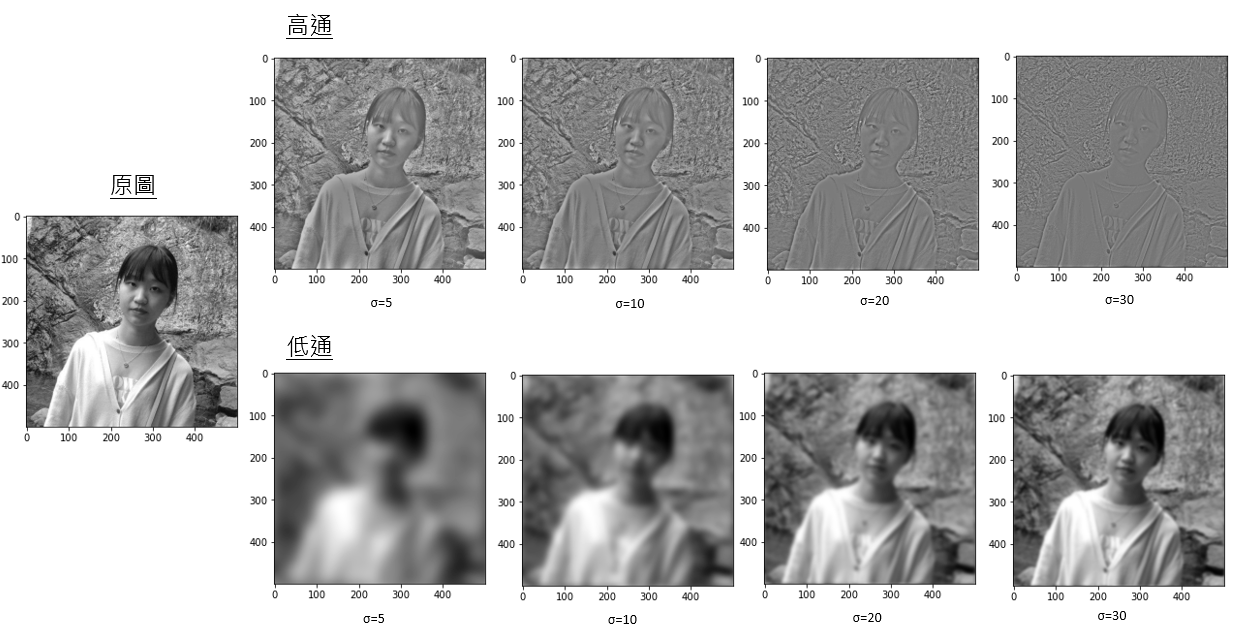### ¶ 4.2 混疊圖片